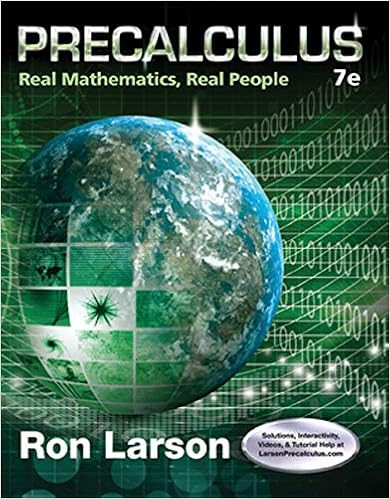# This study resource was shared via courseherocom

• Test Prep
• 9
• 100% (1) 1 out of 1 people found this document helpful

This preview shows page 5 - 9 out of 9 pages.

##### We have textbook solutions for you!
The document you are viewing contains questions related to this textbook.The document you are viewing contains questions related to this textbook.
Chapter 6 / Exercise 6
Precalculus: Real Mathematics, Real People
LarsonExpert Verified
This study resource was shared via CourseHero.com
##### We have textbook solutions for you!
The document you are viewing contains questions related to this textbook.The document you are viewing contains questions related to this textbook.
Chapter 6 / Exercise 6
Precalculus: Real Mathematics, Real People
LarsonExpert Verified
Problem 2 (25 pts.): A block of mass M = 5.4 kg, at rest on a horizontal frictionless table, is attached to a rigid support by a spring of constant k = 6000 N/m which is initially uncompressed. A bullet of mass m = 9.5 g and velocity v of magnitude 630 m/s strikes and becomes embedded in the block. This compresses the spring and sets block and spring in simple harmonic motion. Determine: a) ( 8 pts. ) the speed of the block immediately after the collision. b) ( 9 pts. ) the amplitude of the harmonic motion. c) ( 8 pts. ) the frequency of the harmonic motion. k m v This study resource was shared via CourseHero.com M
Problem 3 (25 pts.) The figure to the right shows a block S with mass M . The block is free to move along a horizontal frictionless surface and connected, by a massless cord that wraps over a frictionless pulley with mass m and radius ( I=mr 2 /2) to a second block H also of mass m . The hanging block H falls as the sliding block S accelerates to the right. a) ( 6 pts.) Draw force diagrams for blocks S and H and the pulley. b) ( 10 pts.) Find the linear acceleration of the rope. c) ( 9 pts.) Find the magnitude of tension in the rope pulling on block S. m a α =-a/r This study resource was shared via CourseHero.com r
Problem 4 (26 pts) As shown in the figure, an overhead view of a thin uniform rod of length 0.80 m and mass M = 1.0 kg rotating horizontally at angular speed 20 rad/s about an axis through its center. A particle of mass M /3 initially attached to one end is ejected from the rod by a small explosion and travels along a straight path that is perpendicular to the rod at the instant of ejection. The particle's final speed v p (after the ejection) is 11.0 m/s. a) ( 6 pts. ) What is the angular momentum (magnitude and direction) of the particle about the center of rod at the moment before the ejection? b) ( 10 pts.) What is the final angular velocity (magnitude and direction) of the rod? c) ( 10 pts. ) What is the change in kinetic energy of the system (the rod plus particle)? This study resource was shared via CourseHero.com
Problem 5 (27 pts.): A block of mass m 1 rests on a frictionless inclined plane at a height above a flat horizontal plane. This mass is released and slides down the incline, eventually colliding elastically with a second block at rest at x 0 with mass m 2 , with m 1 = 3 m 2 /4. Immediately after the elastic collision, block number 2 moves to the right with velocity v 2f . It encounters a region that has friction with a coefficient of kinetic friction of μ k , traveling a distance d before coming to rest. Answer the following questions about these two blocks. a) ( 7 pts.) Determine an equation for v 2f immediately after the collision (using m 2 , h , and b) ( 7 pts.) Determine an equation for d (in terms of m 2 , h , μ k , and g ), the distance that block 2 travels on the friction surface, before coming to a stop. c) ( 6 pts.) What is the magnitude (equation) and sign of the work done by the frictional force on block 2? d) ( 7 pts. ) What is the velocity v f of block 1 after the collision (in terms of m 2 , h , and g ). Use vector notation. This study resource was shared via CourseHero.com h = g ) 1 Powered by TCPDF ()
•••